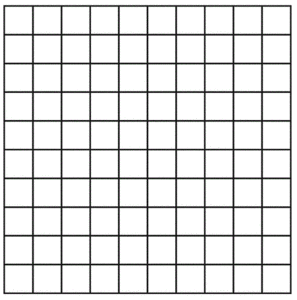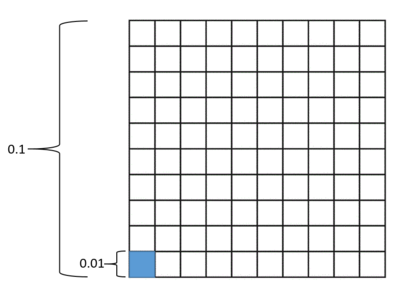# Tenths of Tenths and Hundredths of Hundredths

Alignments to Content Standards: 6.NS.B.3

A large square has both sides subdivided into $10$ equal pieces.If the large square has a side length of $100$ units, then the side length of a small square is $100\div10=10$ units. In this case, the area of the large square is $100\times100 = 10,000$ square units and the area of a small square is $10\times10 = 100$ square units.

1. What if the side length of the large square is $10$? What is the area of the large square? What is the area of a single small square?
2. What if the side length of the large square is $1$? What is the area of the large square? What is the area of a single small square?
3. How can you see $0.1 \times 0.1$ in the diagram? What is $0.1 \times 0.1$?
4. Show $0.01 \times 0.01$ in the diagram, and find this product. Explain how you can see $0.01 \times 0.01$ in your diagram.

## IM Commentary

The purpose of this task is to relate what students know about multiplication, area, and fractions to multiplying decimals for powers of ten that are less than 1. The questions are carefully sequenced so that students are lead to construct an argument for why, from a geometric perspective, $0.1\times0.1$ is 0.01 and $0.01\times0.01$ is 0.0001. Eventually, students should generalize their understanding and know that the number of decimal places in a product is the same as the total number of decimal places in the factors. This task gives a geometric basis for understanding why that is true.

There are two ways of thinking about the area of a small square, and both support reasoning about the product of decimals. You can think of the area of a small square as the product of its side lengths, and you can also think of the area of a small square as $1/100$ the area of the large square. If students are surprised by how tiny the numbers get for the area of a small square, it is helpful to point out that a small square is only $1/100$ the area of the large square.

Only one copy of the grid is provided in the task statement, but it would be helpful to provide students with several copies of the grid to draw on. Here's an editable student page for use with the task. Alternatively, a full-page copy of a grid could be placed in a sheet protector so that students can draw on it with a dry erase marker.

## Solution

1. If the side length of the large square is $10$, then the area of the large square is $10 \times 10 = 100$. The side length of a small square is $1$, so the area of a small square is $1 \times 1 = 1$. We could also reason that the area of a small square is $\frac{1}{100}$ of the area of the large square, so the area of a small square is $100 \div 100 = 1$.
2. When the side length of the large square is $1$, the area of the large square is $1$. A small square is $\frac{1}{100}$ of the large square, so the area of a small square is $\frac{1}{100}$ of $1$, or $0.01$.
3. We can also write the area of the small square as the product of its side lengths. The side length of a small square is $1 \div 10 = 0.1$, and $0.1\times0.1$ is $0.01$.
4. The area of the large square in the diagram below is $0.01$ (we know this from part b). The area of a small square is $\frac{1}{100}$ the area of the large square, or $0.01 \div 100$ and is also the same as $0.01 \times 0.01$. The product is $0.0001$.